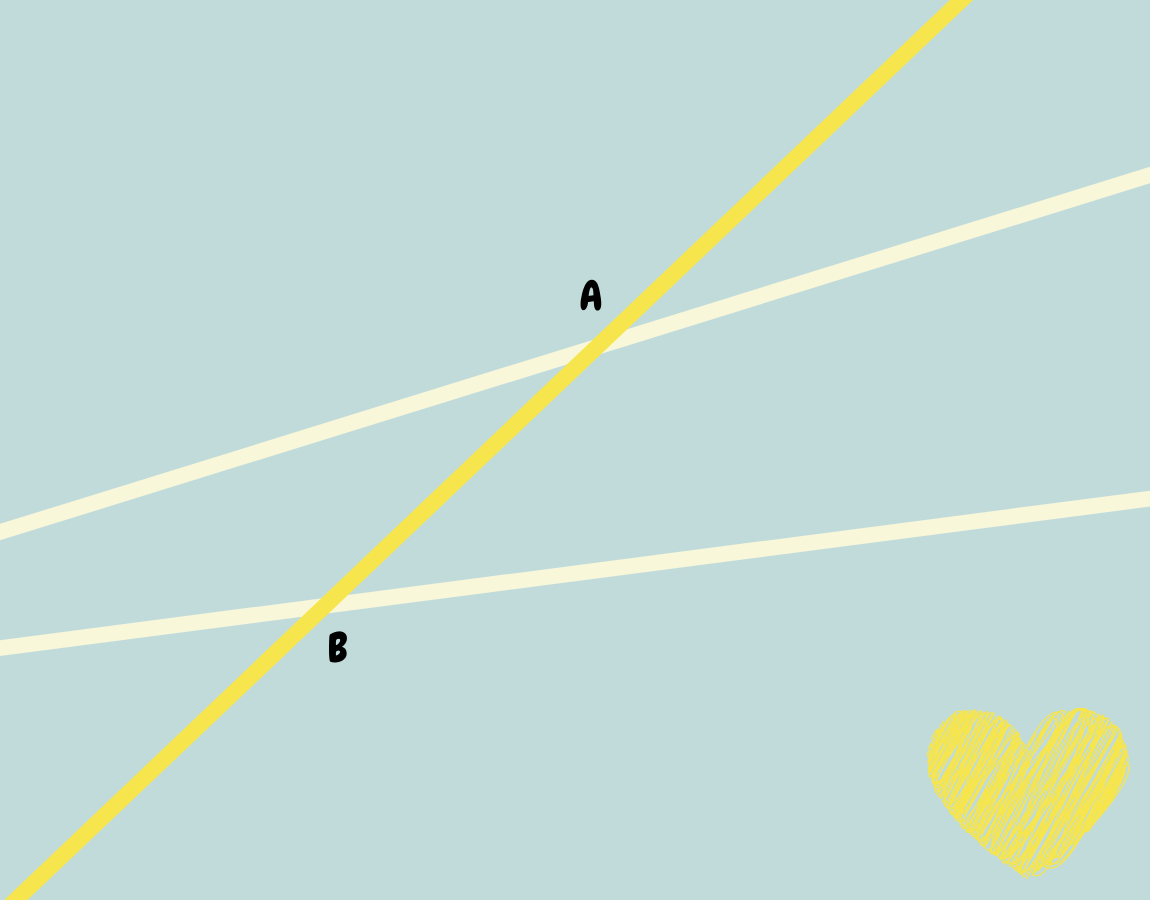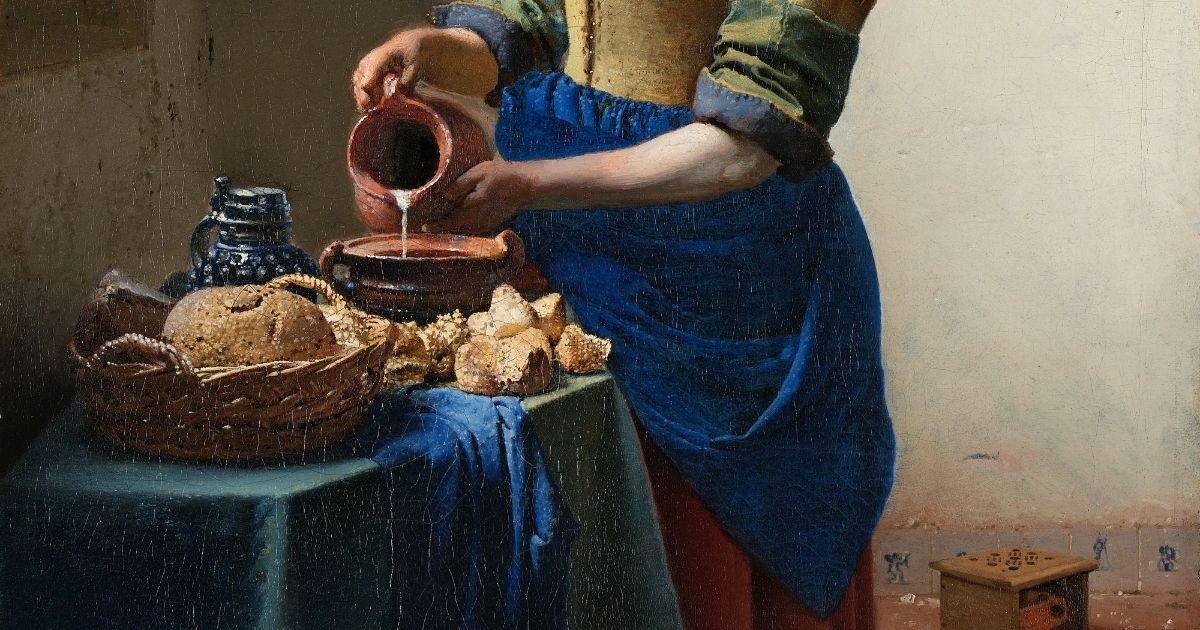Hunter's Woods PH

# Montessori Geometry: Two Straight Lines Crossed by a Transversal

## An introduction to two straight lines (not necessarily parallel) crossed by a transversal. Illustrates interior, exterior, alternate, consecutive, and corresponding angles.

In this presentation, the two straight lines crossed by a transversal are not necessarily parallel. It is an introduction to the terminology and aims to arrive at two conclusions:

1. Two straight lines cut by a transversal will form eight angles.
2. Two straight lines cut by a transversal form four interior and four exterior angles.

## Presentation: Two Straight Lines Crossed by a Transversal

### Definitions

A transversal is a line that passes through two lines in the same plane at two distinct points.

Alternate angles are pairs of angles that:

• have distinct vertex points,
• lie on opposite sides of the transversal, and
• are either:
• both interior (called alternate interior angles), or
• both exterior (called alternate exterior angles).

Consecutive interior angles are pairs of angles that:

• have distinct vertex points,
• lie on the same side of the transversal, and
• are both interior.

Corresponding angles are pairs of angles that:

• have distinct vertex points,
• lie on the same side of the transversal, and
• one angle is interior while the other is exterior.

## Mastery Quiz: Two Straight Lines Crossed by a Transversal

Name The Angles## Trending Quizzes### Dino Quiz! Level 1 Challenge### Greek Mythology Quiz: Can You Identify These Greek Gods and Goddesses?### How Well Do You Know Our Solar System?